# poctave

Generate octave spectrum

## Syntax

``p = poctave(x,fs)``
``p = poctave(xt)``
``p = poctave(pxx,fs,f)``
``p = poctave(___,type)``
``p = poctave(___,Name,Value)``
``[p,cf] = poctave(___)``
``[p,cf,t] = poctave(___)``
``poctave(___)``

## Description

example

````p = poctave(x,fs)` returns the octave spectrum of a signal `x` sampled at a rate `fs`. The octave spectrum is the average power over octave bands as defined by the ANSI S1.11 standard . If `x` is a matrix, then the function estimates the octave spectrum independently for each column and returns the result in the corresponding column of `p`.```
````p = poctave(xt)` returns the octave spectrum of a signal stored in the MATLAB® timetable `xt`.```

example

````p = poctave(pxx,fs,f)` performs octave smoothing by converting a power spectral density, `pxx`, to a 1/b octave power spectrum, where b is the number of subbands in the octave band. The frequencies in `f` correspond to the PSD estimates in `pxx`.```

example

````p = poctave(___,type)` specifies the kind of spectral analysis performed by the function. Specify `type` as `'power'` or `'spectrogram'`.```

example

````p = poctave(___,Name,Value)` specifies additional options for any of the previous syntaxes using name-value arguments.```
````[p,cf] = poctave(___)` also returns the center frequencies of the octave bands over which the octave spectrum is computed.```
````[p,cf,t] = poctave(___)` additionally returns a time vector, `t`, corresponding to the center times of the segments used to compute the power spectrum estimates when `type` is `'spectrogram'`.```
````poctave(___)` with no output arguments plots the octave spectrum or spectrogram in the current figure. If `type` is specified as `'spectrogram'`, then this function is supported only for single-channel input.```

## Examples

collapse all

Generate ${10}^{5}$ samples of white Gaussian noise. Create a signal of pseudopink noise by filtering the white noise with a filter whose zeros and poles are all on the positive x-axis. Visualize the zeros and poles.

```N = 1e5; wn = randn(N,1); z = [0.982231570015379 0.832656605953720 0.107980893771348]'; p = [0.995168968915815 0.943841773712820 0.555945259371364]'; [b,a] = zp2tf(z,p,1); pn = filter(b,a,wn); zplane(z,p)```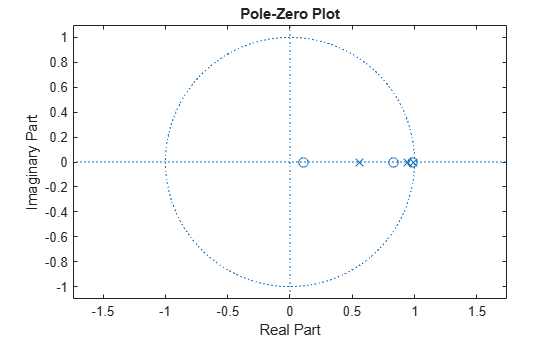Create a two-channel signal consisting of white and pink noise. Compute the octave spectrum. Assume a sample rate of 44.1 kHz. Set the frequency band from 30 Hz to the Nyquist frequency.

```sg = [wn pn]; fs = 44100; poctave(sg,fs,'FrequencyLimits',[30 fs/2]) legend('White noise','Pink noise','Location','SouthEast')```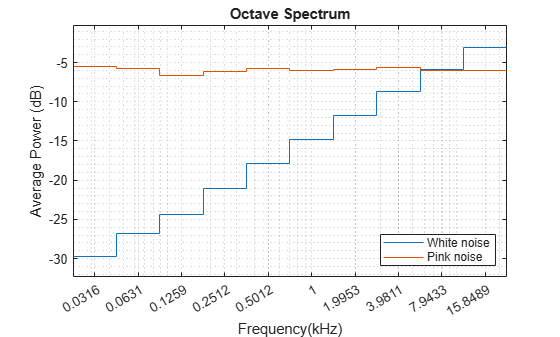The white noise has an octave spectrum that increases with frequency. The octave spectrum of the pink noise is approximately constant throughout the frequency range. The octave spectrum of a signal illustrates how the human ear perceives the signal.

Generate ${10}^{5}$ samples of white Gaussian noise sampled at 44.1 kHz. Create a signal of pink noise by filtering the white noise with a filter whose zeros and poles are all on the positive x-axis.

```N = 1e5; fs = 44.1e3; wn = randn(N,1); z = [0.982231570015379 0.832656605953720 0.107980893771348]'; p = [0.995168968915815 0.943841773712820 0.555945259371364]'; [b,a] = zp2tf(z,p,1); pn = filter(b,a,wn);```

Compute the Welch estimate of the power spectral density for both signals. Divide the signals into 2048-sample segments, specify 50% overlap between adjoining segments, window each segment with a Hamming window, and use 4096 DFT points.

`[pxx,f] = pwelch([wn pn],hamming(2048),1024,4096,fs);`

Display the spectral densities over a frequency band ranging from 200 Hz to the Nyquist frequency. Use a logarithmic scale for the frequency axis.

```pwelch([wn pn],hamming(2048),1024,4096,fs) ax = gca; ax.XScale = 'log'; xlim([200 fs/2]/1000) legend('White','Pink')```Compute and display the octave spectra of the signals. Use the same frequency range as in the previous plot. Specify six bands per octave and compute the spectra using 8th-order filters.

```poctave(pxx,fs,f,'BandsPerOctave',6,'FilterOrder',8,'FrequencyLimits',[200 fs/2],'psd') legend('White','Pink')```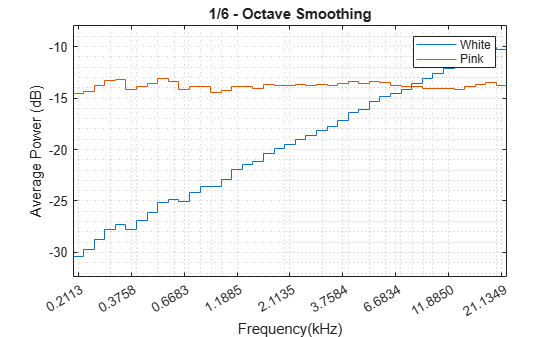Read an audio recording of an electronic toothbrush into MATLAB®. The toothbrush turns on at about 1.75 seconds and stays on for approximately 2 seconds.

`[y,fs] = audioread('toothbrush.m4a');`

Compute the octave spectrogram of the audio signal. Specify 48 bands per octave and 82% overlap. Restrict the total frequency range from 100 Hz to `fs`/2 Hz and use C-weighting.

`poctave(y,fs,'spectrogram','BandsPerOctave',48,'OverlapPercent',82,'FrequencyLimits',[100 fs/2],'Weighting','C')`Generate ${10}^{5}$ samples of white Gaussian noise sampled at 44.1 kHz. Create a signal of pink noise by filtering the white noise with a filter whose zeros and poles are all on the positive x-axis.

```N = 1e5; fs = 44.1e3; wn = randn(N,1); z = [0.982231570015379 0.832656605953720 0.107980893771348]'; p = [0.995168968915815 0.943841773712820 0.555945259371364]'; [b,a] = zp2tf(z,p,1); pn = filter(b,a,wn);```

Compute the octave spectrum of the signal. Specify three bands per octave and restrict the total frequency range from 200 Hz to 20 kHz. Store the name-value pairs in a cell array for later use. Display the spectrum.

```flims = [200 20e3]; bpo = 3; opts = {'FrequencyLimits',flims,'BandsPerOctave',bpo}; poctave(pn,fs,opts{:});```Compute the octave spectrum of the signal with the same settings, but use C-weighting. The C-weighted spectrum falls off at frequencies above 6 kHz.

```hold on poctave(pn,fs,opts{:},'Weighting','C')```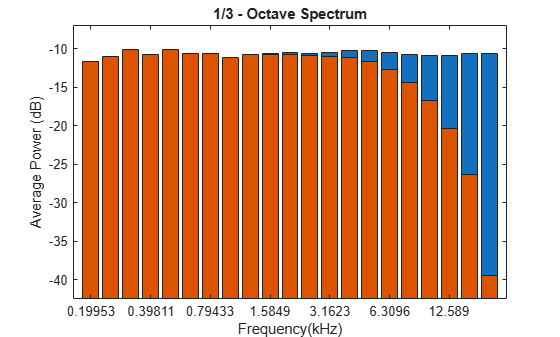Compute the octave spectrum again, but now use A-weighting. The A-weighted spectrum peaks at about 3 kHz and falls off above 6 kHz and at the lower end of the frequency band.

```poctave(pn,fs,opts{:},'Weighting','A') hold off legend('Pink noise','C-weighted','A-weighted','Location','SouthWest')```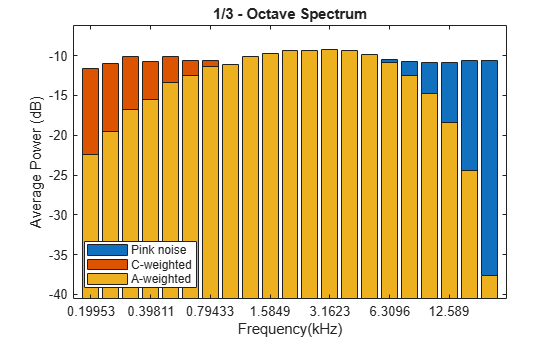## Input Arguments

collapse all

Input signal, specified as a vector or matrix. If `x` is a vector, then `poctave` treats it as a single channel. If `x` is a matrix, then `poctave` computes the octave spectrum or spectrogram independently for each column and returns the result in the corresponding column of `p`. If `type` is set to `'spectrogram'`, the function concatenates the spectrograms along the third dimension of `p`.

Example: `sin(2*pi*(0:127)/16)+randn(1,128)/100` specifies a noisy sinusoid.

Example: `[2 1].*sin(2*pi*(0:127)'./[16 64])` specifies a two-channel sinusoid.

Data Types: `single` | `double`

Sample rate, specified as a positive scalar expressed in hertz. The sample rate cannot be lower than 7 Hz.

Input timetable. `xt` must contain increasing, finite, uniformly spaced row times. If `xt` represents a multichannel signal, then it must have either a single variable containing a matrix or multiple variables consisting of vectors.

If a timetable has missing or duplicate time points, you can fix it using the tips in Clean Timetable with Missing, Duplicate, or Nonuniform Times.

Example: `timetable(seconds(0:4)',randn(5,1))` specifies a random process sampled at 1 Hz for 4 seconds.

Power spectral density (PSD), specified as a vector or matrix with real nonnegative elements. The power spectral density must be expressed in linear units, not decibels. Use `db2pow` to convert decibel values to power values. If `type` is `'spectrogram'`, then each column in `pxx` is considered to be the PSD for a particular time window or sample.

Example: ```[pxx,f] = periodogram(cos(pi./[4;2]*(0:159))'+randn(160,2))``` specifies the periodogram PSD estimate of a noisy two-channel sinusoid sampled at 2π Hz and the frequencies at which it is computed.

PSD frequencies, specified as a vector. `f` must be finite, strictly increasing, and uniformly spaced in the linear scale.

Example: ```[pxx,f] = periodogram(cos(pi./[4;2]*(0:159))'+randn(160,2))``` specifies the periodogram PSD estimate of a noisy two-channel sinusoid sampled at 2π Hz and the frequencies at which it is computed.

Type of spectrum to compute, specified as `'power'` or `'spectrogram'`.

• `'power'` — Compute the octave power spectrum of the input.

• `'spectrogram'` — Compute the octave spectrogram of the input. The function divides the input into segments and returns the short-time octave power spectrum of each segment.

### Name-Value Arguments

Specify optional comma-separated pairs of `Name,Value` arguments. `Name` is the argument name and `Value` is the corresponding value. `Name` must appear inside quotes. You can specify several name and value pair arguments in any order as `Name1,Value1,...,NameN,ValueN`.

Example: `'Weighting','A','FilterOrder',8` computes the octave spectrum using A-weighting and 8th-order filters.

Number of subbands in the octave band, specified as `1`, `3/2`, `2`, `3`, `6`, `12`, `24`, `48`, or `96`. This parameter dictates the width of a fractional-octave band. In such a frequency band, the upper edge frequency is the lower edge frequency times 21/b, where b is the number of subbands.

Data Types: `single` | `double`

Order of bandpass filters, specified as a positive even integer.

Data Types: `single` | `double`

Frequency band, specified as an increasing two-element vector expressed in hertz. The lower value of the vector must be at least 3 Hz. The upper value of the vector must be smaller than or equal to the Nyquist frequency. If the vector does not contain an octave center, `poctave` may return a center frequency outside the specified limits. To ensure a stable filter design, the actual minimum achievable frequency limit increases to `3*fs/48e3` if the sample rate exceeds 48 kHz. If this argument is not specified, `poctave` uses the interval ```[max(3,3*fs/48e3) fs/2]```.

Data Types: `single` | `double`

Frequency weighting, specified as one of these:

• `'none'``poctave` does not perform any frequency weighting on the input.

• `'A'``poctave` performs A-weighting on the input. The ANSI S1.42 standard defines the A-weighting curve. The IEC 61672-1 standard defines the minimum and maximum attenuation limits for an A-weighting filter. The ANSI S1.42.2001 standard defines the weighting curve by specifying analog poles and zeros.

• `'C'``poctave` performs C-weighting on the input. The ANSI S1.42 standard defines the C-weighting curve. The IEC 61672-1 standard defines the minimum and maximum attenuation limits for a C-weighting filter. The ANSI S1.42.2001 standard defines the weighting curve by specifying analog poles and zeros.

• Vector — `poctave` treats the input as a vector of coefficients that specify a finite impulse response (FIR) filter.

• Matrix — `poctave` treats the input as a matrix of second-order section coefficients that specify an infinite impulse response (IIR) filter. The matrix must have at least two rows and exactly six columns.

• 1-by-2 cell array — `poctave` treats the input as the numerator and denominator coefficients, in that order, that specify the transfer function of an IIR filter.

• `digitalFilter` object — `poctave` treats the input as a filter that was designed using `designfilt`.

This argument is supported only when the input is a signal. Octave smoothing does not support frequency weighting.

Example: `'Weighting',fir1(30,0.5)` specifies a 30th-order FIR filter with a normalized cutoff frequency of 0.5π rad/sample.

Example: `'Weighting',[2 4 2 6 0 2;3 3 0 6 0 0]` specifies a third-order Butterworth filter with a normalized 3-dB frequency of 0.5π rad/sample.

Example: `'Weighting',{[1 3 3 1]/6 [3 0 1]/3}` specifies a third-order Butterworth filter with a normalized 3-dB frequency of 0.5π rad/sample.

Example: `'Weighting',designfilt('lowpassiir','FilterOrder',3,'HalfPowerFrequency',0.5)` specifies a third-order Butterworth filter with a normalized 3-dB frequency of 0.5π rad/sample.

Data Types: `single` | `double` | `char` | `string` | `cell`

Lower bound for nonzero values, specified as a real scalar. The function sets those elements of `p` such that 10 log10(`p`) ≤ `'MinThreshold'` to zero. Specify `'MinThreshold'` in decibels.

Data Types: `single` | `double`

Length of data segments, specified as a nonnegative integer. `'WindowLength'` must be less than or equal to the length of the input signal. If not specified, the length of data segments is calculated based on the size of the input signal. This input is valid only when `type` is `'spectrogram'`.

Data Types: `single` | `double`

Overlap percent between adjoining segments, specified as a real scalar in the interval [0, 100). If not specified, `'OverlapPercent'` is zero. This input is valid only when `type` is `'spectrogram'`.

Data Types: `single` | `double`

## Output Arguments

collapse all

Octave spectrum or spectrogram, returned as a vector, matrix, or 3-D array. The third dimension, if present, corresponds to the input channels.

Center frequencies, returned as a vector. `cf` contains a list of center frequencies of the octave bands over which `poctave` estimated the octave spectrum. `cf` has units of hertz.

Center times, returned as a vector. If the input is a PSD, then `t` represents the sample indices corresponding to the columns of `pxx`. This argument applies only when `type` is `'spectrogram'`.

## Algorithms

collapse all

Octave analysis is used to identify sound or vibration levels across a broad frequency range in a process that resembles how a human ear perceives sound. The signal spectrum is split into octave or fractional-octave bands. The frequency limit of each band is twice the lower frequency limit, thus the bandwidth increases at higher frequencies.

### Using Octave Filters

To perform octave analysis, the `poctave` function creates a filter bank of parallel bandpass filters. Each digital bandpass filter is mapped to an equivalent Butterworth lowpass analog filter . The analog filter is mapped back to a digital bandpass filter using a bandpass version of the `bilinear` transformation, and the result is returned as a cascade of fourth-order sections.

The lower and upper edge frequencies of each octave band are given by

`${f}_{l}=cf\cdot \left({G}^{-1/2b}\right)$`

`${f}_{u}=cf\cdot \left({G}^{1/2b}\right)$`

where fc is the center frequency of each band defined by the ANSI S1.11-2004 standard  and returned in `cf`, G is a conversion constant (${10}^{3/10}$), and b is the number of bands per octave.

For more information on the design and implementation of octave filters, see Digital Filter Design (Audio Toolbox).

### Using Octave Smoothing

The `poctave` function calculates the average power over each octave band by integrating the power spectral density (PSD) of the signal within the band using the rectangle method. The average power of an octave band represents the signal level at the band center frequency.

• When a band edge falls within a bin, the function assigns to the band only the fraction of power corresponding to the percentage of the frequency bin that the band occupies. For example, this diagram shows an octave band whose edges fall within two different frequency bins, represented by orange and blue dashed rectangles. The power within the shaded regions is computed for the given octave band.• When a band edge falls at 0 or at the Nyquist frequency, fNyquist, the function assigns to the band two times the fraction of power corresponding to the percentage of the frequency bin that the band occupies. This duplication accounts for the half bin power that is present in the range [–w/`2`, `0`] and [fNyquist, fNyquist + w/`2`], where w is the bin width. For example, this diagram shows an octave band whose right edge falls at the Nyquist frequency. The power within the shaded region is computed for the given octave band.Smith, Julius Orion, III. "Example: Synthesis of 1/F Noise (Pink Noise)." In Spectral Audio Signal Processing. https://ccrma.stanford.edu/~jos/sasp/.

 Specification for Octave-Band and Fractional-Octave-Band Analog and Digital Filters. ANSI Standard S1.11-2004. Melville, NY: Acoustical Society of America, 2004.

 Orfanidis, Sophocles J. Introduction to Signal Processing. Englewood Cliffs, NJ: Prentice Hall, 2010.